Checkout JEE MAINS 2022 Question Paper Analysis : Checkout JEE MAINS 2022 Question Paper Analysis :

# Visualising Solid Shapes

Visualising solid shapes explained here students of class 7 and 8 to understand the view of solid figures. Plane figures or shapes have two measurements namely length and breadth. Hence, they are called two-dimensional shapes whereas a solid object has three measurements namely length, breadth, height or depth. Hence, they are called three-dimensional shapes. Also, a solid shape occupies some space. Two-dimensional and three-dimensional figures can also be briefly named as 2-D and 3-D figures, respectively.

## Two-dimensional and Three -dimensional Shapes

Some two-dimensional figures are shown below.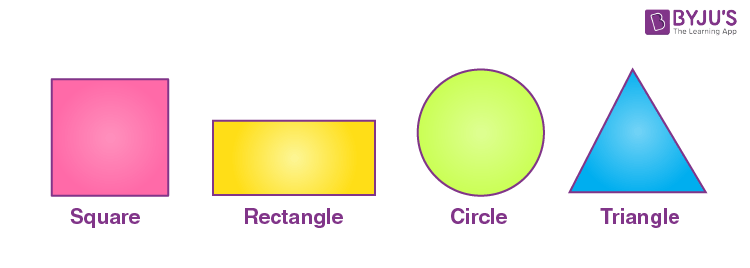Some three-dimensional figures are shown below: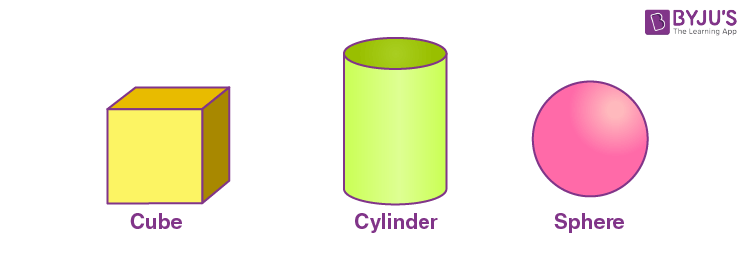Three-dimensional figures have different numbers of faces, edges and vertices. All the flat surfaces of the figure are called faces, these faces are 2-D shapes. The line segment where the faces of three-dimensional figures meet each other is called the edge of the shape. The corners or points where the edges of the figure meet each other are called vertices (if one such point is there, then it is called a vertex).

## Parts of Solid Shapes

Consider a cuboid, the faces, edges and vertices are shown below: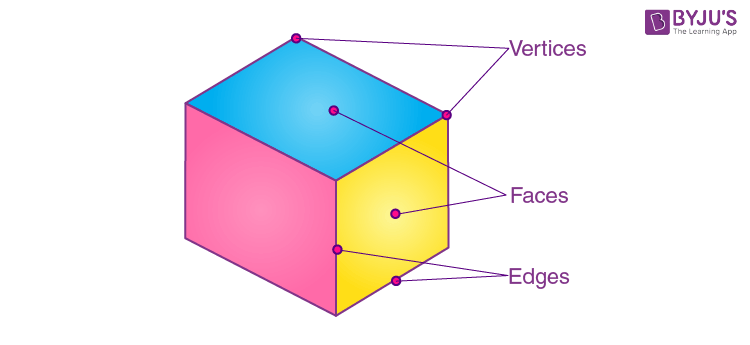The number of faces = 6

Number of edges = 12

Number of vertices = 8

Three-dimensional shapes are further classified into two types.

1. Polyhedrons (or regular polyhedrons)
2. Three-dimensional figures with curved surfaces

### Polyhedrons, Prism and Pyramid

 Name Shape Properties Cube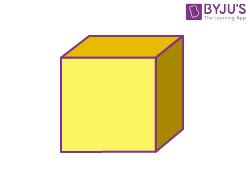Faces = 6 Edges = 12 Vertices =8 Cuboid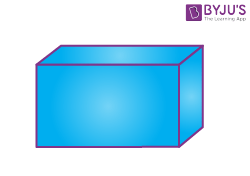Faces = 6 Edges = 12 Vertices =8 Prism (Triangular prism)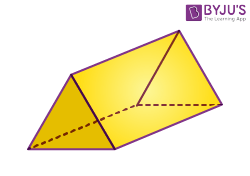Faces = 5 Edges = 9 Vertices = 6 Pyramid (square based pyramid)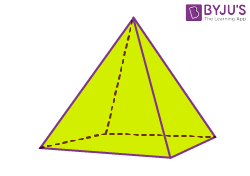Faces = 5 Edges = 8 Vertices = 5 Triangular pyramid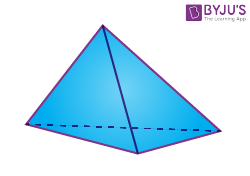Faces = 4 Edges = 6 Vertices = 4

### Three-dimensional figures with curved faces

 Name Shape Properties Cylinder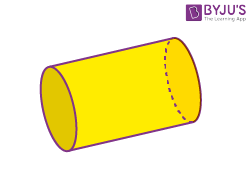Faces = 3 (curved faces = 1, places surfaces =2) Edges = 2 Vertices = 0 ConeFaces = 2 (curved face = 1, plane surface = 1) Edges = 2 Vertices = 1 Sphere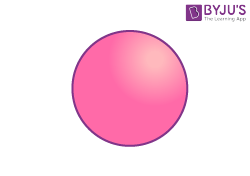Faces = 1 (curved surface) Edges = 0 Vertices = 0

## Nets for 3-D shapes

A two-dimensional shape that can be folded to form a three-dimensional shape or a solid is referred to as a geometry net. When the surface of a three-dimensional shape is laid out flat showing each face of the shape, the obtained pattern is called a net. A solid may have different nets. In simple words, a net is an unfolded form of a 3D figure.

 Shape Solid Net Cube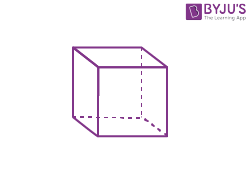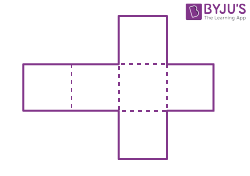Cuboid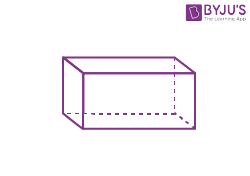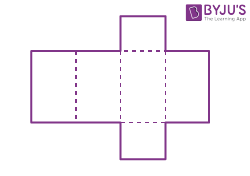Triangular prism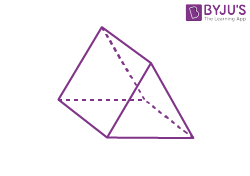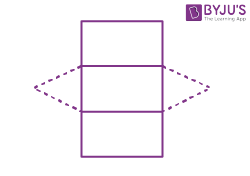Square pyramid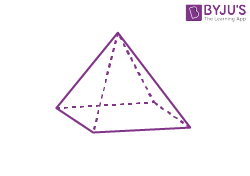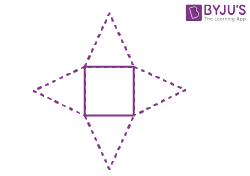Triangular pyramid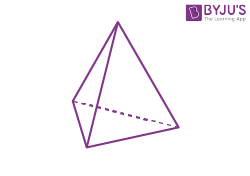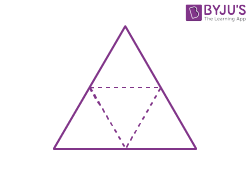Cylinder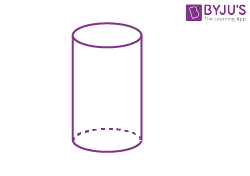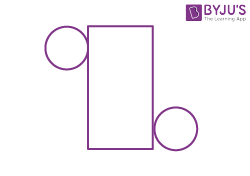Cone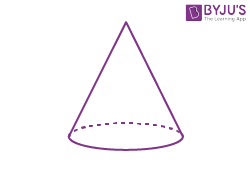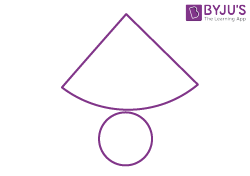## Video Lesson on Nets of Solids

#### To Know About Nets Of Solid Shapes, Watch The Below Video: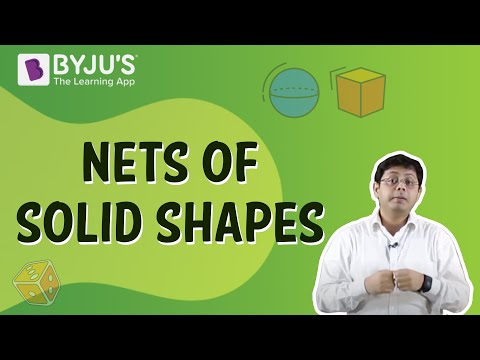## Visualising solid shapes Examples

A three-dimensional object or shape can look differently from different positions (or sides) so they can be drawn from different perspectives, this is called visualising a solid shape. For example, views of a hut and a solid with three cubes from different sides are given below.

Example 1: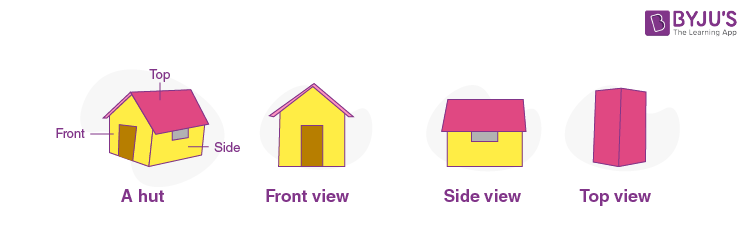As shown in the above, the front view of a hut is the combination of a square with a conical top, side view and top view is the combination of two rectangular surfaces.

Example 2: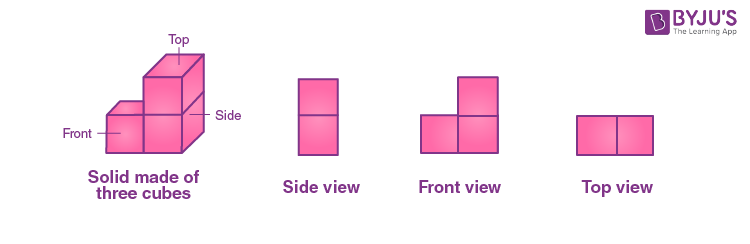In the above figure, one side of a solid has two square surfaces which are vertically connected, front view gas three square surfaces in L shape and top view has two square surfaces which are horizontally connected.

## Related Articles

Find questions and solutions for visualising solid shapes concepts in the below given links.

Test your Knowledge on Visualising Solid Shapes